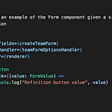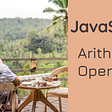# What is the Math Library?

JavaScript has a built-in Math library that I found very helpful many times already (refer to a few of my algorithm review problems). Math is a built-in object, not a function. It has properties and methods of static mathematical constants and functions.

1. Math.ceil(x)
This method rounds a number (usually a decimal) upwards to its nearest integer and returns the result. If the number x is an integer then there will be nothing to round.
`Math.ceil(1.4) // -> 2Math.ceil(2) // -> 2`
2. Math.floor(x)
This method is the opposite of Math.ceil(x). It rounds the decimal number downwards to the nearest integer and returns the result. `Math.floor(1.6) // -> 1Math.floor(1) // -> 1`
3. Math.PI
This is a property that returns the value of pi (3.14159….). This helps whenever you’re trying to calculate the circumference of a circle when it’s ever necessary. I used to be obsessed with the value of pi when I was a kid so I enjoy this property existing.
4. Math.pow(x,y)
This method returns the value of x to the power of y. We are also able to use the ** operator to do the same thing. The issue with ** operand is that it isn’t compatible with Internet Explorer. Math.pow(x,y) gives us cross-browser support.
`Math.pow(2,3) // -> 82 ** 3 // -> 8`
5. Math.sign(x)
This method checks if a number is negative, positive, or zero. If it’s negative, it returns -1. If it’s positive, it returns 1. If it’s zero, it returns 0. I found this method really useful on one of my previous LeetCode algorithm problems.
`Math.sign(3) // -> 1Math.sign(-5) // -> -1Math.sign(0) // -> 0`

# Happy Coding!!

--

--

--

## More from Ajak Cyer

Software Engineering Student at Flatiron NYC. Learning day by day 💻

Love podcasts or audiobooks? Learn on the go with our new app.

## Eval(“console.log(‘RCE Warning’)”)## How to Create Your Own NPM Package## Tales from the bryptjs library: Cryptography and Securing Passwords## Build a Vuex Module## How Experienced Programmers Code Quickly## Out with the Old, In with the New## Introducing React-Forms-Processor## Ajak Cyer

Software Engineering Student at Flatiron NYC. Learning day by day 💻

## JavaScript Arithmetic Operators## Why a coding bootcamp? Why Flatiron?## Javascript for Kids## What is THIS? Understanding the execution context in JavaScript